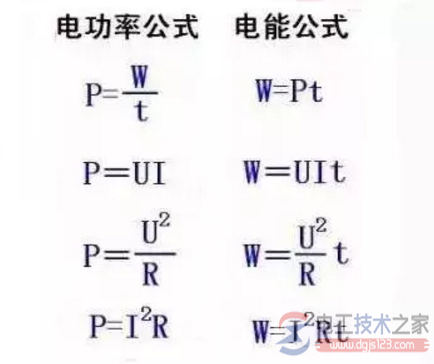`>`首页 > 电工基础 > 电工入门`>`电力换算公式_电能计算公式_电工常用单位换算公式 正文

# 电力换算公式_电能计算公式_电工常用单位换算公式

###### 来源：电工天下 编辑：小电工发布时间：2020-04-28 15:27:05W表示功，功的单位是：焦耳（J）

W=P*t

P---功率（单位：瓦w）

t---时间（单位：秒s）

W=U*I*t

U---电压（单位：伏V）

I---电流（单位：安A）

t---时间（单位：秒s）

W=I^2*R*t

I---电流（单位：安A）

R---电阻（单位：欧Ω）

t---时间（单位：秒s）

W=U^2/R*t

U---电压（单位：伏V）

R---电阻（单位：欧Ω）

t---时间（单位：秒s）

P表示功率，功率单位是：瓦特(W)

P=W/t

W---电功（单位：焦j或千瓦时kWh）

t---时间（单位：秒s）

P=U*I

U---电压（单位：伏V）

I---电流（单位：安A）

P=U^2/R（只能用于纯电阻电路）

U---电压（单位：伏V）

R---电阻（单位：欧Ω）

P=I^2*R（只能用于纯电阻电路）

I---电流（单位：安A）

R---电阻（单位：欧Ω）

Q表示电荷，电荷的单位是：库仑(C)

I表示电流，电流的单位是：安培（A）

J表示电流密度，电流的单位是：安培/方米A/㎡

1MV=1000KV=1000000V=1000000000mV=1000000000000μV

U表示电压，电压单位是：伏特（V）

U=I*R

I---电流（单位：安A）

R---电阻（单位：欧Ω）

U=P/I

P---功率（单位：瓦w）

I---电流（单位：安A）

U=I*ρ*L/S

I---电流（单位：安A）

ρ---电阻率（单位：欧姆·米Ω·m）

L---物体长度（单位：米m）

S---物体的截面面积（单位：平方米㎡）

F表示电容，电容的单位是：法拉（F）

1KΩ=1000Ω

R表示电阻，电阻的单位是：欧姆（Ω）

R=U/I

U---电压（单位：伏V）

I---电流（单位：安A）

R=ρ*L/S

ρ---电阻率（单位：欧姆·米Ω·m）

L---物体长度（单位：米m）

S---物体的截面面积（单位：平方米㎡）

G表示物体的电导，电导的单位是：西门子（S）或者欧姆（Ω）

G=1/R

R---电阻（单位：欧Ω）

G=I/U

I---电流（单位：安A）

U---电压（单位：伏V）

ρ表示电阻率，电阻率单位是：欧姆·米(Ω·m)

ρ=1/κ

κ---电阻率（单位：西门子/米S/m）

ρ=R*S/L

S---横截面积（单位：平方米㎡）

R---电阻（单位：欧Ω）

L---导线的长度（单位：米m）

ρ=E/J

E---电场强度(单位：牛/库N/C)

J---电流密度(单位：安/平方米A/㎡)

ρ=ρo*(1+a*t)

ρo---0℃时的电阻率（单位：欧姆·米Ω·m）

t---摄氏温度(单位：摄氏度)

a---电阻率温度系数

κ（希腊字母不是字母K）表示电导率，电导率的单位是：西门子/米（S/m）

κ=1/ρ

ρ---电阻率（单位：欧姆·米Ω·m）

L表示电感，电感的单位是：亨利（H）

X表示电抗，电抗的单位是：欧姆（Ω）

X=XLXc

XL---电路的感抗

Xc---电路的容抗

XL=ωL

L---电感（单位：亨利H）

XL=2*π*f*L

f---频率（单位：赫兹Hz）

L---电感（单位：亨利H）

Xc=1/（ω*C）

C---电容（单位：法拉F）

Xc=1/（2*π*f*C）

f---频率（单位：赫兹Hz）

C---电容（单位：法拉F）

ω=2*π*f

f---频率（单位：赫兹H）

L(mH)=(0.08D.D.N.N)/(3D+9W+10H)

D------线圈直径

N------线圈匝数

d-----线径

H----线圈高度

W----线圈宽度

l=(0.01*D*N*N)/(L/D+0.44)

l=25330.3/[(f0*f0)*c]

1、针对环行CORE，有以下公式可利用:(IRON)

L=N2．ALL=电感值（H)

H-DC=0.4πNI/lN=线圈匝数(圈)

AL=感应系数

H-DC=直流磁化力I=通过电流(A)

l=磁路长度（cm)

l及AL值大小，可参照Micrometal对照表。例如:以T50-52材，线圈5圈半，其L值为T50-52(表示OD为0.5英吋)，经查表其AL值约为33nH

L=33．(5.5)2=998.25nH≒1μH

H-DC=0.4πNI/l=0.4×3.14×5.5×10/3.74=18.47（查表后）

2、介绍一个经验公式

L=(k*μ0*μs*N2*S)/l

μ0为真空磁导率=4π*10(-7)。（10的负七次方）

μs为线圈内部磁芯的相对磁导率，空心线圈时μs=1

N2为线圈圈数的平方

S线圈的截面积，单位为平方米

l线圈的长度，单位为米

k系数，取决于线圈的半径（R)与长度(l)的比值。

I----电流（A）, R---电阻（Ω).

U、I---分别为相电压、电流.

P=√3UIcosφ 式中：U、I---分别为线电压、线电流.

cosφ ---功率因数,若为三相阻性负载,如三相电炉, cosφ=1

U1：U2=R1：R2

W1：W2=R1：R2=U1：U2

P1：P2=R1：R2=U1：U2

I1：I2=R2：R1

W1：W2=I1：I2=R2：R1

P1：P2=R2：R1=I1：I2

⑶同一用电器的电功率

①额定功率比实际功率等于额定电压比实际电压的平方 Pe/Ps=(Ue/Us)的平方

2．有关电路的公式

⑴电阻 R

①电阻等于材料密度乘以（长度除以横截面积） R=密度×（L÷S）

②电阻等于电压除以电流 R=U÷I

③电阻等于电压平方除以电功率 R=UU÷P

⑵电功 W

⑶电功率 P

①电功率等于电压乘以电流 P=UI

②电功率等于电流平方乘以电阻 P=IIR（纯电阻电路）

③电功率等于电压平方除以电阻 P=UU÷R(同上)

④电功率等于电功除以时间 P＝W：T

⑷电热 Q

⑴串联电路 P（电功率）U（电压）I（电流）W（电功）R（电阻）T（时间）

U1：U2=R1：R2

W1：W2=R1：R2=U1：U2

P1：P2=R1：R2=U1：U2

⑵并联电路

I1：I2=R2：R1

W1：W2=I1：I2=R2：R1

P1：P2=R2：R1=I1：I2

⑶同一用电器的电功率

①额定功率比实际功率等于额定电压比实际电压的平方 Pe/Ps=(Ue/Us)的平方

2．有关电路的公式

⑴电阻 R

①电阻等于材料密度乘以（长度除以横截面积） R=密度×（L÷S）

②电阻等于电压除以电流 R=U÷I

③电阻等于电压平方除以电功率 R=UU÷P

⑵电功 W

⑶电功率 P

①电功率等于电压乘以电流 P=UI

②电功率等于电流平方乘以电阻 P=IIR（纯电阻电路）

③电功率等于电压平方除以电阻 P=UU÷R(同上)

④电功率等于电功除以时间 P＝W：T

⑷电热 Q

P=1.732×（380×I×COSΦ）是三相电路中星型接法的感性负载功率计算公式

= 3*相电压U*相电流I（角形接法）

= 3*相电压U*相电流I*功率因数COSΦ（角形接法）

I----电流（A）， R---电阻（Ω).

U、I---分别为相电压、电流。

P=√3UIcosφ 式中：U、I---分别为线电压、线电流。

cosφ ---功率因数，若为三相阻性负载，如三相电炉， cosφ=1

1A（安）=1000mA（毫安）=1000000μA（微安

= 3*相电压U*相电流I（角形接法）

U=IR,I=U/R,R=U/I,

P=UI,I=P/U,U=P/I

P=U2/R,R=U2/P

`相关文章`